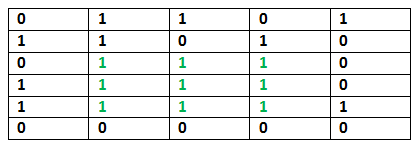# Java Program for Maximum size square sub-matrix with all 1s

• Last Updated : 12 Dec, 2018

Given a binary matrix, find out the maximum size square sub-matrix with all 1s.

For example, consider the below binary matrix.## Java

 `// JAVA Code for Maximum size square``// sub-matrix with all 1s``public` `class` `GFG {``    ``// method for Maximum size square sub-matrix with all 1s``    ``static` `void` `printMaxSubSquare(``int` `M[][])``    ``{``        ``int` `i, j;``        ``int` `R = M.length; ``// no of rows in M[][]``        ``int` `C = M[``0``].length; ``// no of columns in M[][]``        ``int` `S[][] = ``new` `int``[R][C];`` ` `        ``int` `max_of_s, max_i, max_j;`` ` `        ``/* Set first column of S[][]*/``        ``for` `(i = ``0``; i < R; i++)``            ``S[i][``0``] = M[i][``0``];`` ` `        ``/* Set first row of S[][]*/``        ``for` `(j = ``0``; j < C; j++)``            ``S[``0``][j] = M[``0``][j];`` ` `        ``/* Construct other entries of S[][]*/``        ``for` `(i = ``1``; i < R; i++) {``            ``for` `(j = ``1``; j < C; j++) {``                ``if` `(M[i][j] == ``1``)``                    ``S[i][j] = Math.min(S[i][j - ``1``],``                                       ``Math.min(S[i - ``1``][j], S[i - ``1``][j - ``1``]))``                              ``+ ``1``;``                ``else``                    ``S[i][j] = ``0``;``            ``}``        ``}`` ` `        ``/* Find the maximum entry, and indexes of maximum entry ``            ``in S[][] */``        ``max_of_s = S[``0``][``0``];``        ``max_i = ``0``;``        ``max_j = ``0``;``        ``for` `(i = ``0``; i < R; i++) {``            ``for` `(j = ``0``; j < C; j++) {``                ``if` `(max_of_s < S[i][j]) {``                    ``max_of_s = S[i][j];``                    ``max_i = i;``                    ``max_j = j;``                ``}``            ``}``        ``}`` ` `        ``System.out.println(``"Maximum size sub-matrix is: "``);``        ``for` `(i = max_i; i > max_i - max_of_s; i--) {``            ``for` `(j = max_j; j > max_j - max_of_s; j--) {``                ``System.out.print(M[i][j] + ``" "``);``            ``}``            ``System.out.println();``        ``}``    ``}`` ` `    ``// Driver program``    ``public` `static` `void` `main(String[] args)``    ``{``        ``int` `M[][] = { { ``0``, ``1``, ``1``, ``0``, ``1` `},``                      ``{ ``1``, ``1``, ``0``, ``1``, ``0` `},``                      ``{ ``0``, ``1``, ``1``, ``1``, ``0` `},``                      ``{ ``1``, ``1``, ``1``, ``1``, ``0` `},``                      ``{ ``1``, ``1``, ``1``, ``1``, ``1` `},``                      ``{ ``0``, ``0``, ``0``, ``0``, ``0` `} };`` ` `        ``printMaxSubSquare(M);``    ``}``}`
Output:
```Maximum size sub-matrix is:
1 1 1
1 1 1
1 1 1
```

Please refer complete article on Maximum size square sub-matrix with all 1s for more details!

My Personal Notes arrow_drop_up